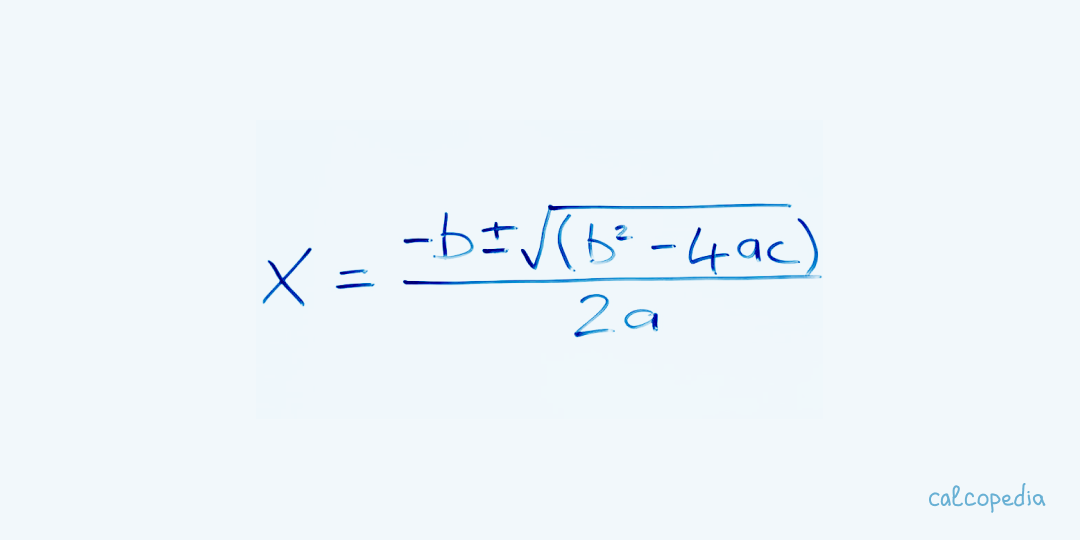Apply methods to solve quadratic equations using formulas, discriminant, and Vieta's theorem.

Result

copy

Contents

## What are Quadratic Equations and How Do We Solve Them?A quadratic equation is a polynomial equation of the second degree. The general form is ax² + bx + c = 0, where a, b, and c are constants, and x represents an unknown variable.

The solutions for x, often known as the roots, can be found using the quadratic formula: x = (-b ± sqrt(b²-4ac)) / 2a

It`s fascinating to note that the term "quadratic" originates from the Latin word "quadratus," meaning square. This is because the degree of the primary term is squared.

The discriminant, represented by the formula b² - 4ac, plays a crucial role in determining the nature of the roots of a quadratic equation. It can be positive, negative, or zero, leading to different types of solutions.

## How to Use the Quadratic Equation Calculator?

With the rise of technology, solving quadratic equations has become a breeze with online calculators. Let`s explore how to use one:

1. Begin by entering the coefficients a, b, and c from your quadratic equation.

2. Ensure that you've entered the correct signs (positive or negative) for each coefficient.

3. Click on the "Calculate" button to start the process.

4. The calculator will display the solutions for x based on the quadratic formula.

5. If the discriminant is positive, you'll receive two distinct real roots. If it`s zero, you'll get one real root. A negative discriminant indicates complex roots.

6. For better understanding, some calculators even provide a graphical representation of the equation.

7. Make sure to double-check your input values if the solutions seem off or unexpected.

## Examples of Calculating Quadratic Equations

Quadratic equations appear in various real-life situations. Let`s explore a few humorous examples:

1. Alien Invasion: If an alien spaceship travels in a path represented by x² - 5x + 6 = 0, find the points where we can intercept them! Solving, we get x = 2 and x = 3. So, set up the defense at these points!

2. Pizza Party: You throw a pizza in the air, and its height as a function of time is given by -16t² + 32t + 5 = 0. Find when the pizza will hit the ground, so you can catch it. The positive root will be the time it takes!

3. Love Life: Your love life`s trajectory is given by x² - 4x + 4 = 0. Find when you'll meet your true love. Spoiler: The roots are real and identical, meaning you've already met them!

## Nuances of Calculating Quadratic Equations

While quadratic equations can be fun, there are certain nuances to keep in mind:

1. Always check the discriminant to determine the nature of the roots.

2. If a = 0, then the equation is not quadratic. It`s linear!

3. Quadratic equations always have two roots, but they can be real or complex.

4. The vertex of the parabola represented by the equation is given by (-b/2a, c - b²/4a).

5. The axis of symmetry is given by x = -b/2a.

6. Complex roots always occur in conjugate pairs.

7. Quadratic equations can represent real-life situations, but ensure the context makes sense with the solutions.

8. Some quadratic equations can be factored easily, offering a quicker solution method.

9. The graph of a positive leading coefficient opens upwards, while a negative one opens downwards.

10. The maximum or minimum value of the quadratic function occurs at the vertex.

### Why are there sometimes two solutions?

Quadratic equations can have up to two distinct roots because of the squared term. Depending on the discriminant, these can be real or complex.

### What if my leading coefficient is zero?

If a = 0, the equation is not quadratic but linear. You won`t need a quadratic formula for that!

### Why do I get complex roots?

Complex roots arise when the discriminant is negative. This means the parabola represented by the equation doesn`t intersect the x-axis.

### Can I always use the quadratic formula?

Yes, the quadratic formula always works for any quadratic equation, but sometimes factoring or completing the square might be easier methods.

### Is the graph always a parabola?

Yes, the graph of a quadratic equation is always a parabola. Its direction (upwards or downwards) depends on the sign of the leading coefficient.

## Similar calculators

You may find the following calculators on the same topic useful:

• Logarithm Calculator. Compute natural, decimal logarithms (or with other bases) online with a solution.
• Raising a Fraction to a Power. Raise any fraction (decimal or common) to any power online.
• Column Multiplication. For simplified multiplication of large numbers, use the traditional column multiplication method.
• Fraction Calculator. Calculate the addition, multiplication, and reduction of regular fractions.
• Roots Calculator. Determine precise values of roots of various degrees, including square and cubic.
• Toss a Coin Online. With this tool, you can toss a coin online as many times as you wish.
• Scientific Calculator. Solve complex equations with our sophisticated (scientific, engineering, financial) calculator.
• Multiplication Table Trainer. Practice and memorize the multiplication table online. Set the multiplier range, and the trainer will create exercises.
• Percentage of a Number Calculator. Calculate online the value of a percentage of any number using this calculator.
• Percentage Calculator. Calculate online the percentage of a number, by how much one number is greater or smaller than another in percentage, or what percentage one number is of another number. Also, add or subtract a percentage from a number.

## Share on social media

If you liked it, please share the calculator on your social media platforms. It`s easy for you and beneficial for the project`s promotion. Thank you!

### Do you have anything to add?

Feel free to share your opinion, comment, or suggestion.